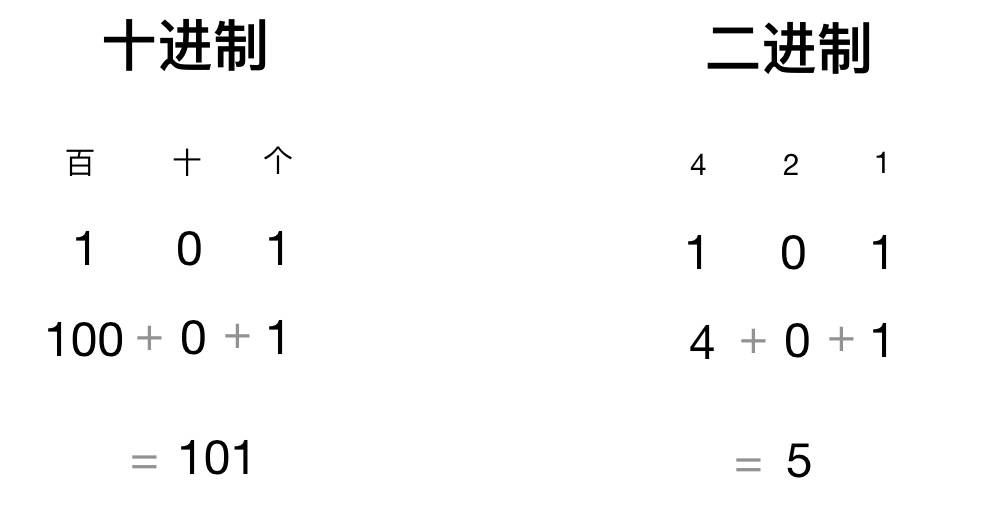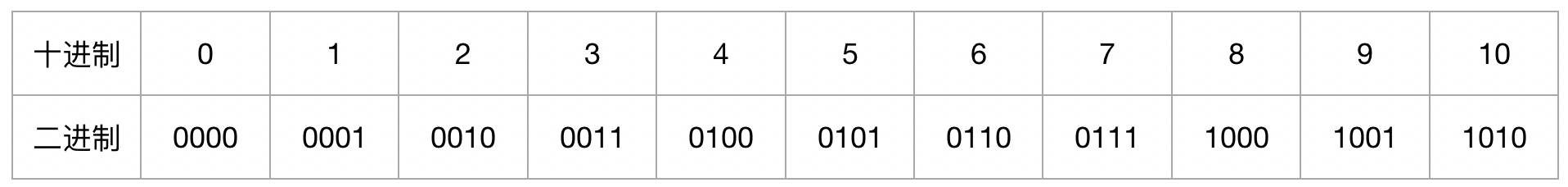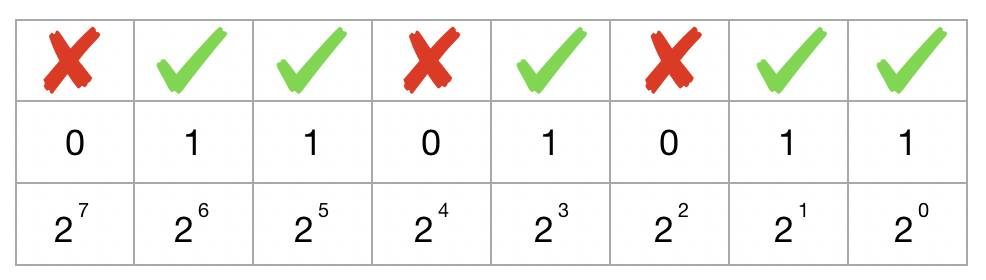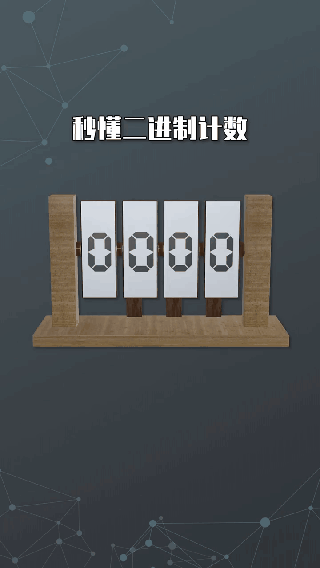# 二进制

## 什么是二进制

100 = 1；

101 = 10；

102 = 100；

103 = 1000；

20 = 1；

21 = 2；

22 = 4；

23 = 8；## 二进制转换

①算出 2 的 n 次幂不大于要表示的值；

②用要表示的值减去 2n，得到剩下的值后，重复步骤 1，直到最后剩下 0 为止。二进制转成十进制就是相反的过程，如 01011001 转成十进制：01011001 = 0 x 27 + 1 x 26 + 0 x 25 + 1 x 24 + 1 x 23 + 0 x 22+ 0 x 21 + 1 x 20 = 89THE END

### 请登录后发表评论请登录后查看评论内容